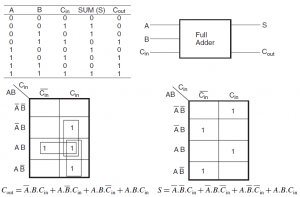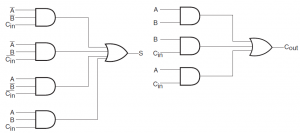Home > combinational logic circuits > full adder

# Full Adder

Full adder is used to add three bits and produce a SUM and a CARRY outputs. Full adder is mainly needed to add large number of bits. e. g. consider the addition of least significant bit (LSB) of two numbers. Here, the sum under of the LSB is recorded and the carry is forwarded to the next bits. Hence, in order to add the next bits, we need to add three bits with carry from previous addition. Same things happens to other bits until the MSB is reached. So full adder is the important component in binary additions. A half-adder can only be used for LSB additions. Figure shows the truth table, K-maps and Boolean expressions for the two output variables, SUM
and CARRY outputs of full adder.Figure below shows the simplified implementation of full adder circuit for both sum and carry.Boolean expression of sum can be implemented by using two-input EX-OR gate in which one of the input is Carry in and the other input is the output of another two-input EX-OR gate with A and B as its inputs. On the other hand Carry output can implemented by ORing the outputs of AND gates.